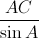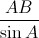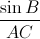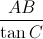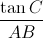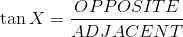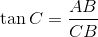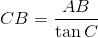# High School Math : Finding Sides

## Example Questions

### Example Question #1 : Solving Triangles

Solve for.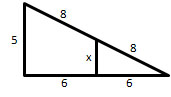(Figure not drawn to scale).

There is not enough information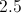Explanation:

The side-angle-side (SAS) postulate can be used to determine that the triangles are similar. Both triangles share the angle farthest to the right. In the smaller triangle, the upper edge has a length of, and in the larger triangle is has a length of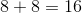. In the smaller triangle, the bottom edge has a length of, and in the larger triangle is has a length of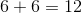. We can test for comparison.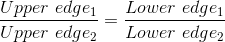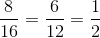The statement is true, so the triangles must be similar.

We can use this ratio to solve for the missing side length.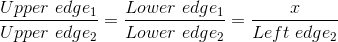To simplify, we will only use the lower edge and left edge comparison.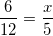Cross multiply.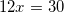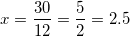### Example Question #2 : Solving Triangles

Solve for.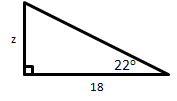(Figure not drawn to scale).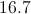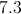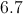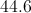Explanation:

We can solve using the trigonometric definition of tangent.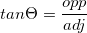We are given the angle and the adjacent side.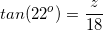We can find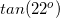with a calculator.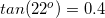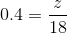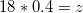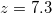### Example Question #3 : Solving Triangles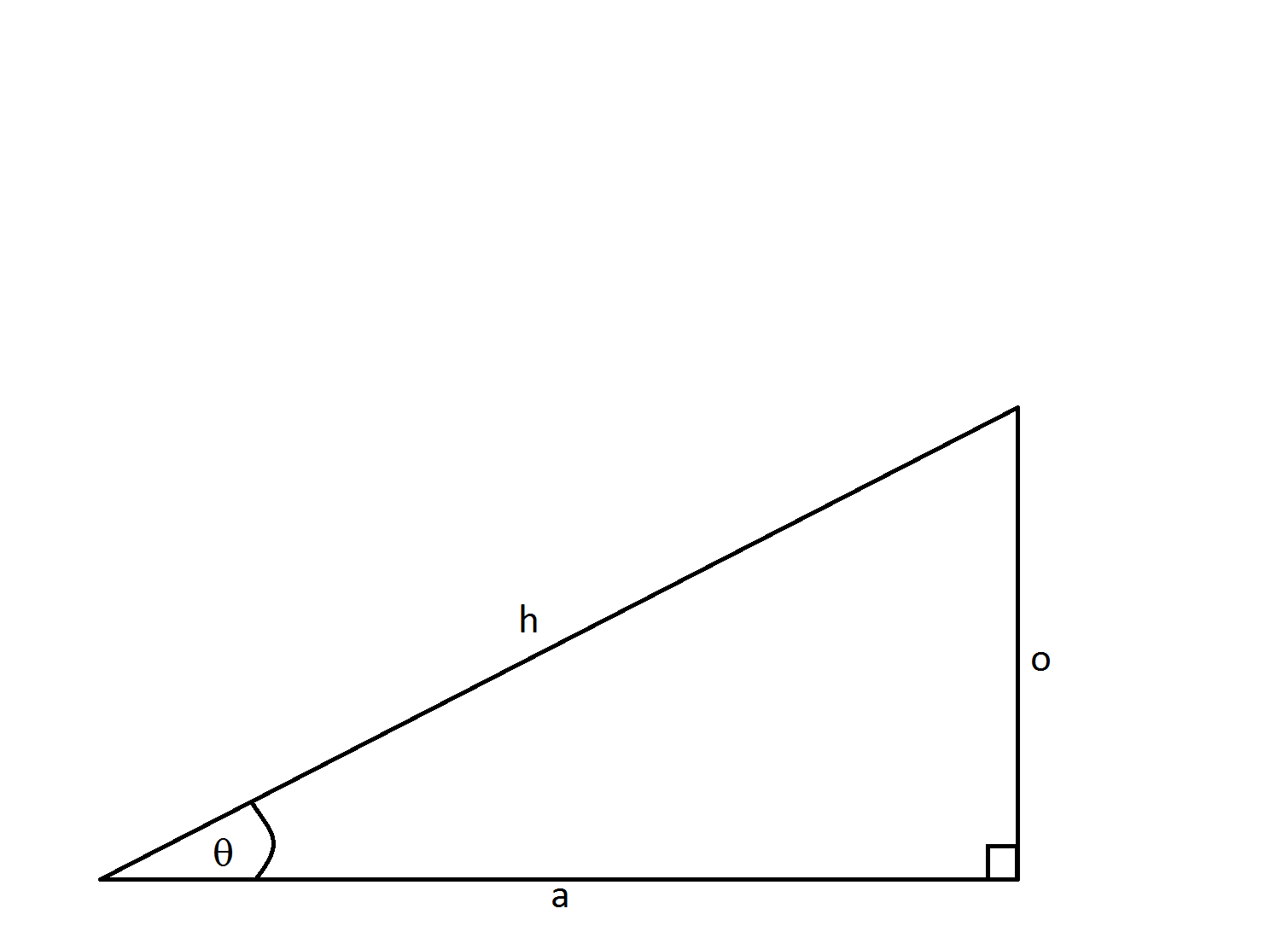If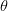equals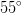and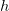is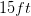, how long is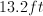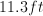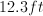Not enough information to solve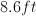Explanation:

This problem can be easily solved using trig identities.  We are given the hypotenuse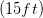and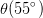.  We can then calculate side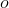using the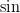.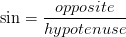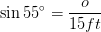Rearrange to solve for.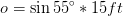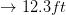If you calculated the side to equal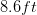then you utilized the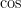function rather than the.

### Example Question #1 : Solving Triangles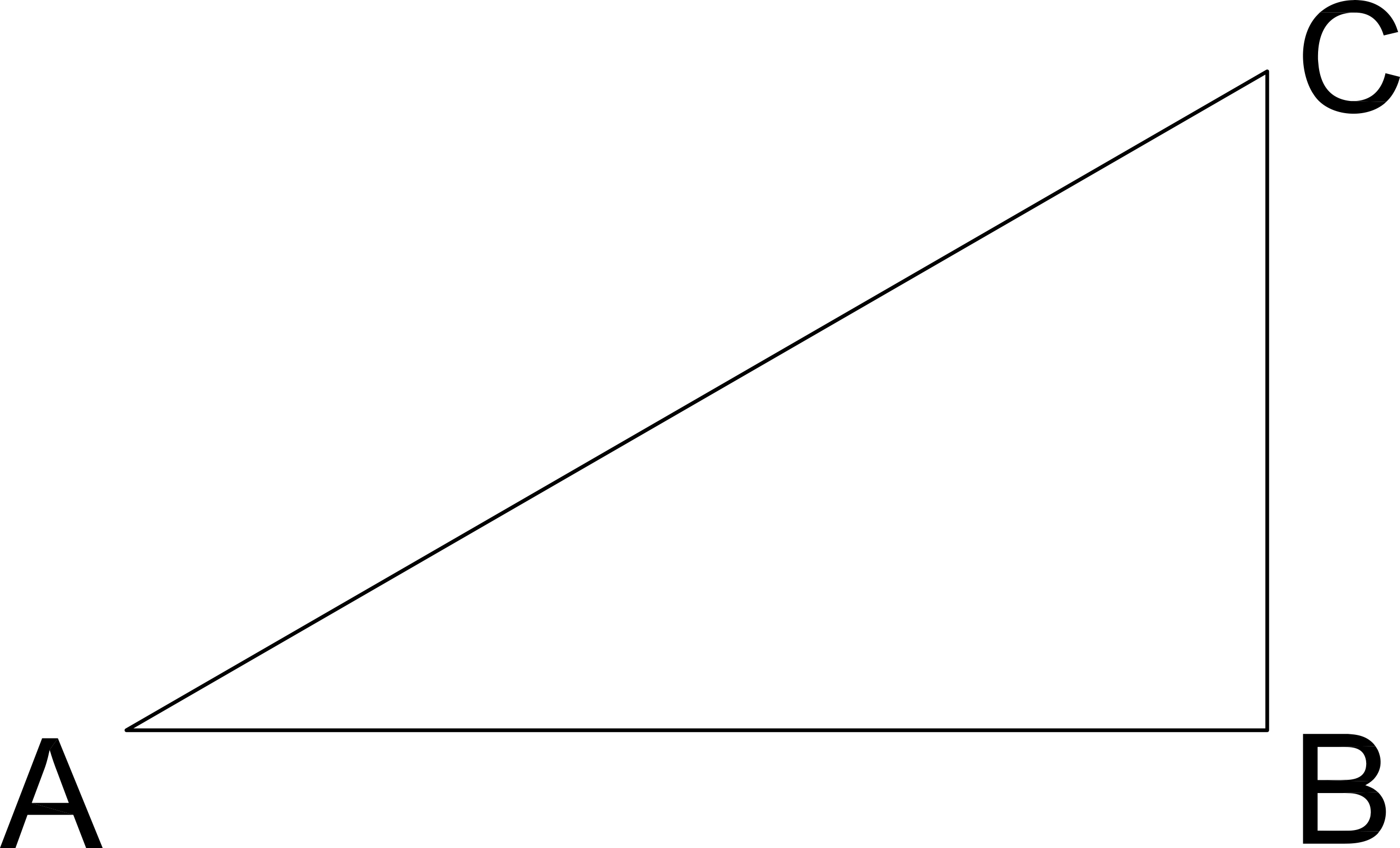What is the length of CB?